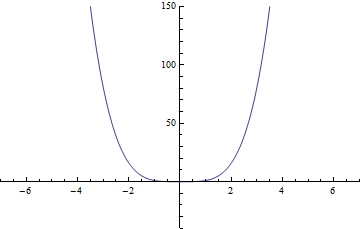# Exporting Data¶

Mathematica has great utilities for exporting data from the application to images, audio files, movies, and more. The image component is the most useful for drafting documents, of course, but others can be useful when applying Mathematica to other problems. For example, if we create an animation (of a dataset through time, or of a simulation), we can easily export it to a format that can be included in a PowerPoint presentation or uploaded to YouTube.

The Export function is surprisingly simple to use, which makes it ever more effective. It is used as Export["filename", graphic, "format"], where the filename is the full path (for ease in finding it later) to the file you want to create, for example “/home/my_user/mathematica_files/picture.png”, graphic is the image (or movie, audio clip, etc.) that you want to save (example to follow), and format is one of the valid file formats (run \$ExportFormats to see which are enabled).

## Exporting Images¶

For a simple example, we have:

Plot[x^2, {x,0,8}]
Export["/home/user/mydirectory/plot.png", %, "PNG"]

which will create a PNG (better resolution than JPG and is still cross-platform) version of the plot and store it in the file specified (which is how each of the images in the Mathematica portion of this textbook have been created).

## Exporting Non-Static Images¶

If we want to export something other than a single static image, we have to update our command slightly. For movies, GIFs, etc. we can simply put the single frames in a list, optionally providing a duration for frames (in seconds):

table = Table[
Plot[x^4 + a x^3 - a^2 x + a, {x, -10, 10},
PlotRange -> {{-7, 7}, {-40, 150}}], {a, 0, 8, .1}];
Export["export_ani.gif", table, "GIF", "DisplayDurations" -> .01]Animated GIF for a polynomial $$x^4+ax^3-a^2x+a;~a\in[0,8]$$.

## Exporting Data¶

If instead of images we want to export data, we can do this as well. For example, we can export a matrix to a CSV (comma-separated values) file. We can even add headers to the data. For example, if we had data=Table[{i, i^2, i^3, Prime[i]}, {i,-20,20}], we can add headers then create the file with:

data=Table[{i, i^2, i^3, Prime[i]}, {i,-20,20}];
PrependTo[data, {"value", "square", "cube", "prime"}];
Export["prime_example.csv", data, "CSV"]


The data is here for reference.

We can export data as well to formats for later use in MATLAB or other languages, and even automatically create archives of multiple files (TAR files, GZIP files, ZIP files). For an updated listing of possible options and examples on how to use each (typically, the single image export is sufficient for any given graph), check out the “Listing of All Formats” page in the Mathematica documentation (Mathematica URL “guide/ListingOfAllFormats”).